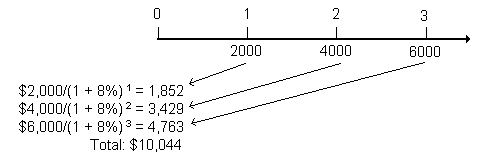Subject 5. The Future Value and Present Value of a Series of Uneven Cash Flows
This subject is for LOS 6.e.

A series of uneven cash flows means that the cash flow stream is uneven over many time periods. There is no single formula available to compute the present or future value of a series of uneven cash flows.

Present Value

When we have unequal cash flows, we must first find the present value of each individual cash flow and then the sum of the respective present values. (This is usually accomplished with the help of a spreadsheet.)

Future Value

Once we know the present value of the cash flows, we can easily apply time-value equivalence by using the formula to calculate the future value of a single sum of money (LOS a).

Example

John wants to pay off his student loan in three annual installments: $2,000,$4,000 and \$6,000, respectively, in the next three years. How much should John deposit into his bank account today if he wants to use the account balance to pay off the loan? Assume that the bank pays 8% interest, compounded annually.If you want to change selection, open original toplevel document below and click on "Move attachment"